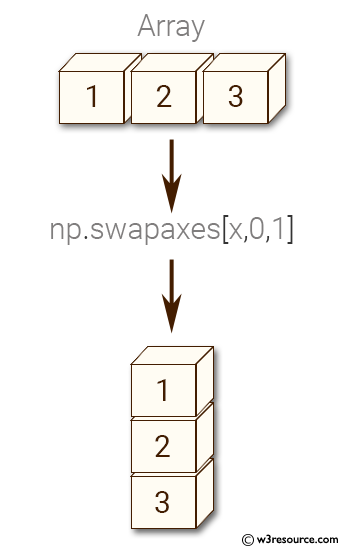﻿ NumPy: Interchange two axes of an array - w3resource# NumPy: Interchange two axes of an array

## NumPy: Array Object Exercise-51 with Solution

Write a NumPy program to interchange two axes of an array.

Pictorial Presentation:Sample Solution:-

Python Code:

``````import numpy as np
x = np.array([[1,2,3]])
print(x)
y =  np.swapaxes(x,0,1)
print(y)
```
```

Sample Output:

```[[1 2 3]]
[

]
```

Python Code Editor:

Have another way to solve this solution? Contribute your code (and comments) through Disqus.

What is the difficulty level of this exercise?

Test your Python skills with w3resource's quiz

﻿

## Python: Tips of the Day

Set comprehension:

```>>> m = {x ** 2 for x in range(5)}
>>> m
{0, 1, 4, 9, 16}
```